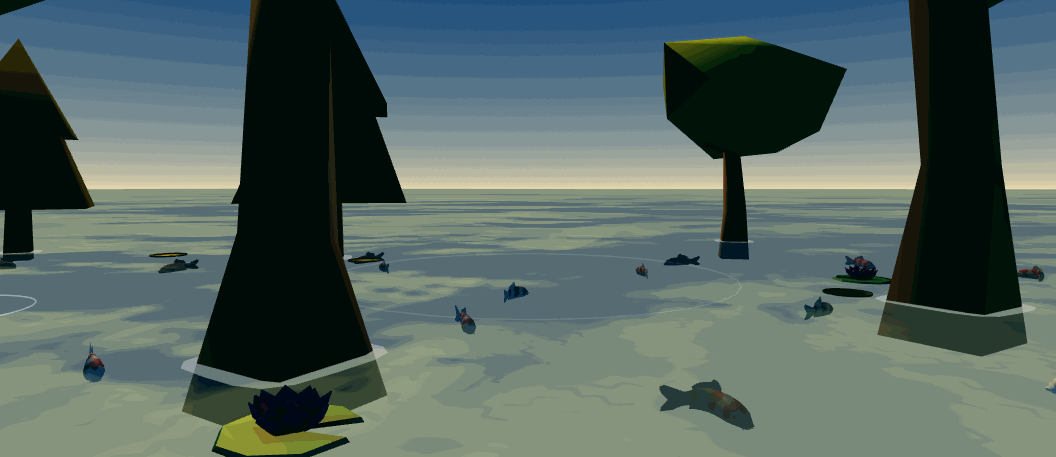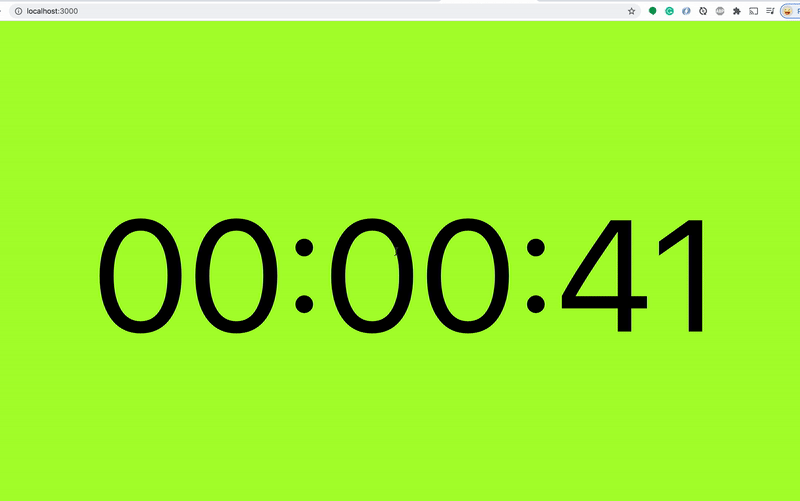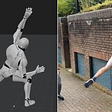This effect is used to make the fish move organically and efficiently in the koi garden demo.

# Curve Modifiers in Three.js

`const canvas = document.querySelector('canvas');const context = canvas.getContext( 'webgl2', { antialias: true } );const renderer = new WebGLRenderer({ canvas, context });`
`import { Flow } from "three/examples/jsm/modifiers/CurveModifier.js";const points = [ new Vector3( 1, 0, z: -1 ), new Vector3( 1, 0, z: 1 ), new Vector3( -1, 0, z: 1 ), new Vector3( -1, 0, z: -1 ),];const curve = new THREE.CatmullRomCurve3(points);curve.curveType = "centripetal";curve.closed = true;const mesh = // some mesh I made earlier;// You may need to tweak the geometry beforehand to get it to// Display with the orientation you expect.mesh.geometry.rotateX( Math.PI );const flow = new Flow( objectToCurve );flow.updateCurve( 0, curve );scene.add( flow.object3D );`
`import { InstancedFlow } from "three/examples/jsm/modifiers/CurveModifier.js";const material = // some materialconst geometry = // some geometryconst curve1 = // A curveconst curve2 = // A curveconst curve3 = // A curveconst curve4 = // A curvegeometry.rotateX( Math.PI );const numberOfInstances = 8;const numberOfCurves = 4;const flow = new InstancedFlow( numberOfInstances, numberOfCurves, geometry, material );// Add the flow object to the scenescene.add( flow.object3D );flow.updateCurve( 0, curve1 );flow.updateCurve( 1, curve2 );flow.updateCurve( 2, curve3 );flow.updateCurve( 3, curve4 );// Do each step below for each numberOfInstances// Set the first instance to be on the first curveflow.setCurve( 0, 0 );// Move the first instance along the curve by a random amountflow.moveIndividualAlongCurve( 0, Math.random() );// Give the first instance a random Colorflow.object3D.setColorAt( 0, new THREE.Color( 0xffffff * Math.random() ) );`

--

--

--

## OOP: From Pseudo-classical class instantiation to Class instantiation## Vue.js or React: What should be your next choice?## How To Create a CountDown Timer In React App## Don’t make them wait — 3 simple ways to make your code go faster## Web Browser … behind the scenes## Top 10 Most Popular JavaScript Projects on GitHub## HOW TO IMPORT SWAGGER FILES TO READYAPI AND POSTMAN?Co-chair of the W3C Immersive Web Working Group, Developer Advocate for Samsung.

## Get started with Augmented Reality on the web using Three.js and WebXR | Part 1## Firebase with Unity (even in WebGL Build!)## Procedural generation of 3d objects with three.js## Capturing Motion